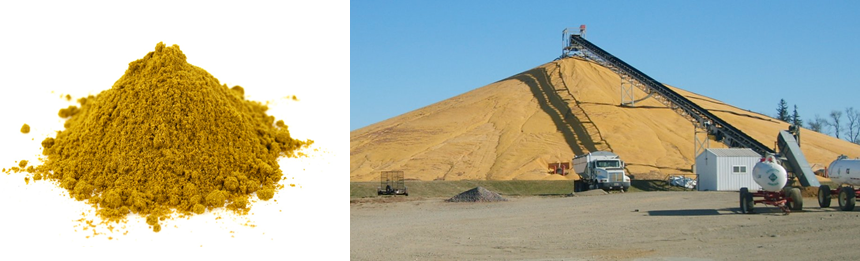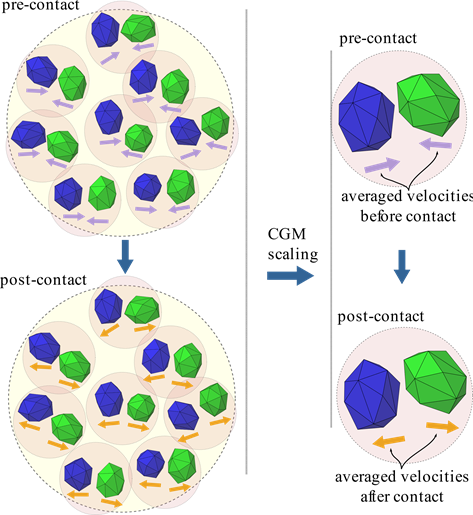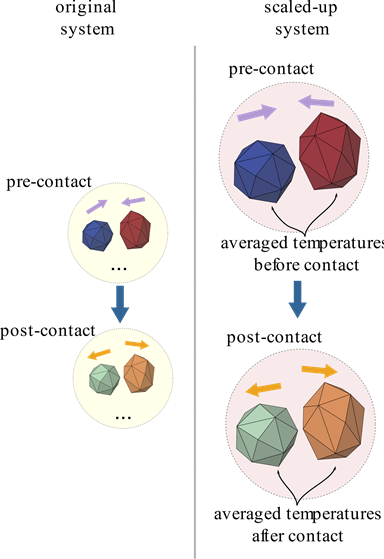## Solve industrial scale simulations with a large number of particles using the Coarse Grain Modeling (CGM)

When using the Discrete Element Method (DEM), all particles inside the domain are tracked (by solving their rotational and translational equations), and this involves solving all the collisions between particles and against boundaries. Therefore, the computational cost of a DEM simulation is proportional to the number of particles (or contacts) in a system.

More efficient DEM codes and faster GPU processing have enabled the simulation of a large number of particles and solving commercial-scale problems is now more attainable than ever. Nowadays, empowered by multi GPU processing, simulations routinely involve solving millions of particles.

However, for many bulk handling processes, it is still unfeasible to run 1:1 simulations for the following reasons:

• For small particles, such as powder, even a small amount of material contains trillions of particles.

• For industrial-scale simulations involving large domains, even for relatively big particles, using the real particle size may lead to quadrillions of particles.

• For non-spherical particles, the accuracy increase obtained by using the real particle shape is counterbalanced by the increased computational cost compared to the simulation using spheres.Scenarios where 1:1 simulations are still unfeasible: (a) a small amount of powder containing trillions of particles. (b) Large industrial-scale simulation containing quadrillions of particles.

## How can CGM help?

One of the strategies often employed to reduce computational cost is by scaling up particle size. CGM (Coarse Grain Modeling) is a method that can be used to reduce the particle number by using larger particles (usually referred to as parcels) to represent groups of the original (smaller) particles, as illustrated in Figure 1.

The scaled-up particle size, Lp, is given by the original particle size, L, multiplied by the scaling factor, fCGM.

Using this model, each parcel represents fCGM3 original particles. That means if a scale factor of 2 is used, the number of particles will be reduced by a factor of 8. Applying a scale factor of 10, the number of particles will be reduced by a factor of 1000.

Moreover, given that the overlap and duration of the collisions are also scaled when increasing the particle size, the CGM approach also allows the usage of larger time steps for the calculations, reducing the execution time.

## There is more to CGM than just increasing the particle size

When scaling-up the system, the main goal is to reduce the number of particles while ensuring that both systems (the original and scaled-up) behave similarly.

The Coarse Grain Model approach used by Rocky DEM follows the procedure defined by Bierwisch et al  to identify modifications that need to be applied to the force and heat transfer models to be used on the scaled-up system. In this scaling approach, simulation of the coarsened particle is performed by adjusting the physical properties or DEM parameters to achieve similarity with the original particle system.

The scaling criteria of each parameter are dependent on the force model employed. Most contact force models implemented in Rocky DEM are self-similar, i.e. requiring no modifications to satisfy the Bierwisch et al. constraint. The correction, when required, is typically the inclusion of a scale-factor multiplying some of the physical properties. For more details about the scaling modifications, check the Rocky DEM Technical manual.

## Similarity criteria for contact models

Essentially, the kinetic energy variation during the collision for the scaled system has to be the same as for the original system. As the effective mass is constant in both, the velocity variation of the scaled-up system must be the same as in the original system.

Figure 2 illustrates this constraint: in the original system (shown on the left side), a certain number of contacts are happening, and the velocities before and after the collisions are depicted by vectors. On the right side of the image, the scaled-up system is shown, in which each group of particles (blue and green) is represented by a single parcel and their velocities, before and after the contact, are given by the average velocity of the particles of the original system.Figure 2. Coarse grain model restriction: the kinetic energy variation during a collision for the original (left) and scaled-up (right) systems should be the same.

## What about the contact heat transfer?

For the contact heat transfer, the constraint is that the temperature variation experienced by two parcels during contact should be the same as two original particles the parcels represent.

Figure 3 illustrates this constraint: in the original system (left side), a number of contacts are happening. One set of particles has a higher temperature (indicated by the red color) and the other set has lower temperatures (indicated by the blue color). During the contact, the particles exchange heat and after the contact, they have closer temperatures (indicated by the lighter colors for each group).

On the scaled-up system (shown on the right), the parcels initially have their temperature defined as the averaged temperature of the original particles they represent. Given the thermal constraint, their temperatures at the end of the contact should be the same as the post-contact averaged temperature of the original particles.Figure 3. Coarse grain model restriction for the contact heat transfer: the temperature variation during a collision for the original (left) and scaled-up (right) systems should be the same.

When accounting for the fluid forces acting on particles, using the CGM model to increase the particle size is critical and deserves an entire post! Stay tuned for an upcoming Rocky blog post to learn more details about this.

## CGM limitations

The particle coarsening should respect the ratio of the particle and the domain size. If the original particle size is much smaller than the characteristic size of the problem, the coarsened particle should still be considerably smaller.

The usage of CGM can underestimate the total energy dissipation on the system due to missed contacts that happen in the original system but not in the scaled model. In an upcoming post, you can find more details about this along with a model that introduces a correction for non-static dense flows with fast collisions.

## Application example

The example below shows a repose/drawdown angle test, commonly used for calibration purposes. The original particle size is 235 μm, and different scaling factors for the CGM model were applied to reduce the computational cost. The gate sliding velocity is 0.5 m/s and the repose and drawdown angles are measured after particles come to rest.

Video 1 shows the behavior of the particles (colored by their initial position) after the gate is opened. It can be seen that, although the number of particles is up to 9x smaller than the original case, the way particles flow is very similar.

Table 1 shows the number of particles as well as the repose and drawdown angles measured for different scaling factors. As expected, the particle scaling using the CGM allowed the system to behave similarly with a considerably smaller number of particles and consequently faster simulations.

#### References

 Bierwisch, C., Kraft, T., Riedel, H., and Moseler, M. (2009). Three-dimensional discrete element models for the granular statics and dynamics of powders in cavity filling. Journal of the Mechanics and Physics of Solids, 6257:10–31

### Lucilla Almeida

CAE Specialist at ESSS, D.Sc.

Lucilla holds a BE (Chemical) undergraduate degree, an M.Sc. in Chemical Engineering and a Ph.D. in Nuclear engineering from the Federal University of Rio de Janeiro. She joined ESSS in 2008 and has spent 5 years focused on applying CFD to solve common engineering problems in the Oil and Gas industry, dealing with turbulent and multiphase flow simulations. Since 2013, she is an Application Engineer for Rocky DEM Business Unit, supporting users, working on consultancy projects and validating models implemented for the CFD-DEM coupling.